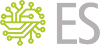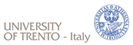MathSAT 5An SMT Solver for Formal Verification & More

SMT-LIBv2 Examples

All the examples can be run like this:

```./mathsat -input=smt2 < FILENAME.smt2
```

Computing models

```;; activate model generation
(set-option :produce-models true)

(declare-fun x () Int)
(declare-fun y1 () Int)
(declare-fun y2 () Int)
(declare-fun z () Int)

(assert (= x y1))
(assert (not (= y1 z)))
(assert (= x y2))
(assert (and (> y2 0) (< y2 5)))

(check-sat)

;; ask for the model value of some of the constants
(get-value (x z))

(exit)
```

Computing unsatisfiable cores

```;; activate unsat core computation
(set-option :produce-unsat-cores true)

(declare-fun x () Int)
(declare-fun y1 () Int)
(declare-fun y2 () Int)
(declare-fun z () Int)

(define-fun A1 () Bool (= x y1))
(define-fun A2 () Bool (not (< z 0)))
(define-fun A3 () Bool (= y1 z))
(define-fun B () Bool (and (= x y2) (not (= y2 z))))

;; use annotation :named to give a name to toplevel formulas. The
;; unsatisfiable core will be a subset of the named formulas. If a toplevel
;; formula doesn't have a name, it will not be part of the unsat core
(assert (! A1 :named First))
(assert (! A2 :named Second))
(assert (! A3 :named Third))
(assert B)

(check-sat)
(get-unsat-core)

(exit)
```

Computing interpolants

```;; activate interpolation
(set-option :produce-interpolants true)

(declare-sort U 0)
(declare-fun x () U)
(declare-fun y1 () U)
(declare-fun y2 () U)
(declare-fun z () U)

(define-fun A1 () Bool (= x y1))
(define-fun A2 () Bool (= y1 z))
(define-fun B () Bool (and (= x y2) (not (= y2 z))))

;; use annotation :interpolation-group to partition the input problem into
;; several groups
(assert (! A1 :interpolation-group g1))
(assert (! A2 :interpolation-group g2))
(assert (! B :interpolation-group g3))

(check-sat)

;; compute an interpolant for the given partition: the argument to
;; get-interpolant is a list of groups forming the A-part of the interpolation
;; problem
(get-interpolant (g1 g2))

(exit)
```

Incremental solving

```;; setting the :global-decls option to true will turn off the scoping of
;; declarations and definitions. According to the SMT-LIBv2 standard,
;; declarations and definitions should be scoped wrt. the current assertion
;; stack. However, sometimes this behaviour is not desirable. MathSAT support
;; both scoped and global declarations (with scoped declarations being the
;; default)
(set-option :global-decls true)

(set-option :produce-models true)

(declare-fun x () Int)
(declare-fun y () Int)

(assert (> x 0)) ;; a permanent constraint
(push 1) ;; push a backtracking point
(assert (< x 0))
(check-sat)

(declare-fun z () Int)
(pop 1)

;; with global declarations, z is still in scope
(assert (= z (+ x 3)))
(push 1)
(assert (< y x))

(declare-fun A () Bool)
(assert (= A (= z (+ y 3))))
(declare-fun B () Bool)
(assert (= B (= x (- 5))))

;; MathSAT supports the (non-standard) command check-sat-assumptions, for
;; checking satisfiability under the given assumptions. At the moment,
;; assumptions can only be Boolean constants. However, arbitrary constraints
;; can be used as assumptions by simply "labeling" them with Boolean
;; constants, as done here
(check-sat-assumptions (A B))

;; for unsatisfiable problems, it is possible to get the set of assumptions
;; responsible for the unsatisfiability, with the get-unsat-assumptions
;; command
(get-unsat-assumptions)

;; the reset-assertions command can be used to remove ALL the assertions (and
;; backtracking points), even those added before any push command
(reset-assertions)
;; the global (> x 0) constraint has been removed, so adding (< x 0) does not
(assert (< x 0))
(check-sat)

;; compute the model value for x
(get-value (x))

(exit)
```

All-Sat support

```(declare-fun x () Int)
(declare-fun y () Int)

(declare-fun a () Bool)
(declare-fun b () Bool)
(declare-fun c () Bool)
(declare-fun d () Bool)

(assert (= (> (+ x y) 0) a))
(assert (= (< (+ (* 2 x) (* 3 y)) (- 10)) c))
(assert (and (or a b) (or c d)))

;; enumerate all the consistent assignments (i.e. solutions) for the given
;; list of predicates. Notice that the arguments to check-allsat can only be
;; Boolean constants. If you need to enumerate over arbitrary theory atoms,
;; you can always "label" them with constants, as done above for
;; "(> (+ x y) 0)", labeled by "a"
(check-allsat (a b))

(exit)
```
Contentsmathsat-announce mailing list